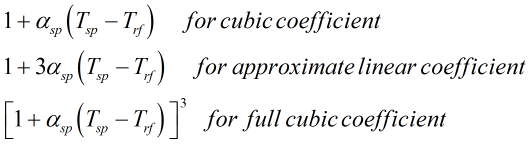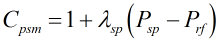# C264 – Flow Rate – Pulse Output Meter

## Description

This calculates the flow rate for pulse output based meters.

Kelton calculation reference C264

KIMS calculation reference K206

Kelton calculation C244 – Calibration Correction

Kelton calculation C248 – Gas Flow Rate Conversion from Qv

Kelton calculation C261 – Gas Flow Rate Conversion from Qm

Kelton calculation C262 – Liquid Flow Rate Conversion

## Options

### Factor Type

• Meter Factor
• K-Factor

This option is used to select the whether a meter factor will be applied to the flow rate calculation. Note that K-factor will always be an input.

### Meter Type

• Volume
• Mass

This option is used to select whether physics of the meter in question infers mass or volume flow rate from its direct outputs.

### Fluid

• Gas
• Liquid

This option is used to select whether the fluid being metered is a liquid or a gas.

### Ctsm

• Not applied
• User entered
• Calculated

This option is used to select whether a correction for temperature on the steel of the meter is made and whether this correction is user entered or calculated.

### Cpsm

• Not applied
• User entered
• Calculated

This option is used to select whether a correction for pressure on the steel of the meter is made and whether this correction is user entered or calculated.

### Interpolation Factor

• Apply

This option is used to apply an interpolation factor.

### Calibration Correction

• Apply

This option is used to apply a calibration correction using C244.

### Spool Temperature Coefficient

• Cubic
• Linear (Approximate)
• Linear (Full)

This option is used to select the temperature coefficient applied for the Ctsm calculation is linear or cubic.

## Calculation

### Correction for temperature on steel of meter

The Ctsm is calculated by:Where αsp = Meter temperature coefficient Tsp = Meter temperature Trf = Meter reference temperature

### Correction for pressure on steel of meter

The Cpsm is calculated by:Where λsp = Meter pressure coefficient Psp = Meter pressure Prf = Meter reference pressure

### Flow Rate

The flow rate, observed volume or mass, is calculated by :Where IntF = Interpolation factor MF = Meter factor f = Frequency KF = K-factor (Will be pulses per volume or pulses per mass depending on whether output flow rate is volumetric or mass based) Ctsm = Correction for temperature on steel of meter Cpsm = Correction for pressure on steel of meter

Note that correction factors are set equal to one if they are not required for the calculation of flow rate.

Back to KIMS Calculations